The QLIM Procedure

Stochastic Frontier Production and Cost Models

Subsections:

Stochastic frontier production models were first developed by Aigner, Lovell, and Schmidt (1977); Meeusen and van den Broeck (1977). Specification of these models allow for random shocks of the production or cost but also include a term for technological or cost inefficiency. Assuming that the production function takes a log-linear Cobb-Douglas form, the stochastic frontier production model can be written as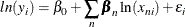where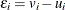. The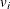term represents the stochastic error component and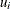is the nonnegative, technology inefficiency error component. Theerror component is assumed to be distributed iid normal and independently from. If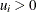, the error term,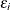, is negatively skewed and represents technology inefficiency. If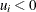, the error termis positively skewed and represents cost inefficiency. PROC QLIM models theerror component as a half normal, exponential, or truncated normal distribution.

The Normal-Half Normal Model

In case of the normal-half normal model,is iid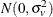,is iid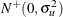withandindependent of each other. Given the independence of error terms, the joint density of v and u can be written as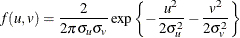Substituting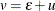into the preceding equation gives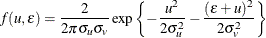Integrating u out to obtain the marginal density function ofresults in the following form: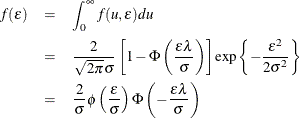where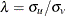and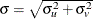.

In the case of a stochastic frontier cost model,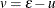and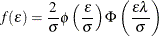The log-likelihood function for the production model with
producers is written as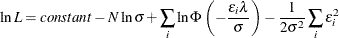The Normal-Exponential Model

Under the normal-exponential model,is iidandis iid exponential with scale parameter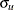. Given the independence of error term componentsand, the joint density of v and u can be written as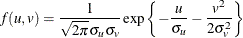The marginal density function offor the production function is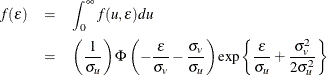and the marginal density function for the cost function is equal to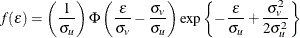The log-likelihood function for the normal-exponential production model withproducers is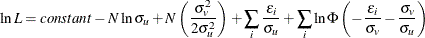The Normal-Truncated Normal Model

The normal-truncated normal model is a generalization of the normal-half normal model by allowing the mean ofto differ from zero. Under the normal-truncated normal model, the error term componentis iidandis iid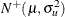. The joint density ofandcan be written as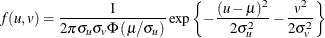The marginal density function offor the production function is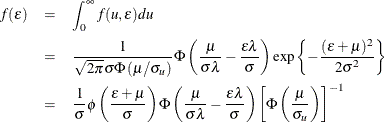and the marginal density function for the cost function is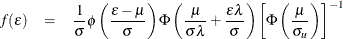The log-likelihood function for the normal-truncated normal production model withproducers is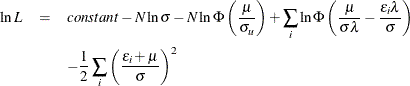For more detail on normal-half normal, normal-exponential, and normal-truncated models, see Kumbhakar and Lovell (2000); Coelli, Prasada Rao, and Battese (1998).